# 2021 HSC Maths Ext 1 Exam Paper Solutions

The 2021 HSC Mathematics Extension (3 Unit) exam paper solutions are here!## 2021 HSC Maths Extension 1 Exam Paper Solutions

Have you seen the 2021 HSC Mathematics Extension (3 Unit) exam paper yet?

In this post, we will work our way through the 2021 HSC Maths Extension 1 (3 Unit) paper and give you the solutions, written by our Head of Mathematics Oak Ukrit and his team. (Doing practice papers? See the solutions for the 2020 HSC Maths Ext 1 Exam here.)

Read on to see how to answer all of the 2021 questions.

## Section 1. Multiple Choice


 Question Answer Solution 1 C \begin{align*} \overrightarrow{PQ} &= \overrightarrow{OQ} – \overrightarrow{OP} \\ &= \binom{2}{5} – \binom{-3}{1}\\ &= \binom{5}{4} \\ \end{align*} 2 B \begin{align*} \text{cos}6x &= 1- 2\text{sin}^23x \\ \text{sin}^2 3x &= \frac{1-\text{cos}6x}{2} \\ ∴ \int \text{sin}^2 3x \ dx &= \int \frac{1 – \text{cos}6x}{2} \ dx \\ \end{align*} 3 D \begin{align*} \text{Use the Remainder Theorem:} \\ P(-2) &= -(-2)^3 -2(-2)^2-3(-2)+8 \\ &= 14 \\ \end{align*} 4 A \begin{align*} \int y \ dy &= \int x \ dx \\ 2 \int y \ dy &= 2 \int x \ dx \\ y^2 &= x^2 + C \\ \end{align*} 5 B \begin{align*} \overrightarrow{OA} · \overrightarrow{OB} =|\overrightarrow{OA}||\overrightarrow{OB}| \text{cos} \theta &< 0 \\ ∴ \text{cos} \theta &< 0 \\ ∴ \theta & \text{ is obtuse}\\ \end{align*} 6 A \begin{align*} r &= 1-P(X=0) \\ &= 1- \binom{10}{0} p^0(1-p)^{10} \\ &= 1 – 0.1^{10} \\ &= 0.9999999999 > 0.9 \\ \end{align*} 7 D \begin{align*} \text{Let } f(x) = -a \text{sin}x + b \text{cos} x &= R \text{cos}(x+ \alpha). \text{ Then:} \\ -a \text{sin} x +b \text{cos} x &= R\text{cos}(x+ \alpha) \\ &= R \text{cos}(\alpha) \text{cos}(x) – R \text{sin}(\alpha)\text{sin}(x) \\ -a \text{sin} x + b \text{cos} x &= -R \text{sin} (\alpha) \text{sin} (x) + R \text{cos} (\alpha) \text{cos} (x) \\ \end{align*}Now, $$R \text{cos} \alpha = b >0$$, $$R \text{sin} \alpha = a >0$$. The signs of the trigonometric ratios indicate that our solution is in the first quadrant, i.e. $$0< \alpha < \frac{pi}{2}$$.Therefore, $$f(x)$$ is a $$\text{cos}(x)$$ graph shifted left by $$\alpha$$.Alternatively, consider the gradient and the sign of $$f(x)$$ at $$x=0$$. $$f(0)=-a \text{sin}(0)+b \text{cos}(0) = b > 0$$, so $$f(x)$$ at $$x=0$$ is positive. $$f'(x)=-a \text{cos}(x) – b\text{sin}(x)$$ and $$f'(0)=-a \text{cos}(0) – b\text{sin}(0)=-a<0$$, so $$f'(x)$$ at $$x=0$$ is decreasing. Therefore, the graph of $$f(x)$$ at $$x=0$$ should be positive and decreasing; this matches (D). 8 C In the domain $$– \frac{ \pi}{2} ≤ t ≤ \frac{ \pi}{2}$$, $$-1 ≤ \text{sin}(t) ≤ 1$$ and $$0 ≤ \text{cos}(t) ≤ 1$$. We note that $$y$$ is always less than the centre 2; so we want $$y = 2 – \text{cos} (t)$$, which is (C). 9 A \begin{align*} \frac{dy}{dx} &= \frac{ \text{cos}x}{ \sqrt{1 – \text{sin}^x}} \\ &= \frac{ \text{cos}x}{|\text{cos} x|} \\ &= \begin{cases} 1 & \text{when } \text{cos}x > 0\\ -1 & \text{when } \text{cos}x < 0\\ \end{cases} \end{align*}As the domain is $$x∈ \bf{R}$$, the solution must be (A).Alternatively, we note that the inner function $$\text{sin} (x)$$ is periodic and has a domain of $$x∈ \bf{R}$$, eliminating (C) and (D). Next, neither the range of $$\text{sin}$$ nor $$\text{sin}^{-1}$$ have any discontinuities, so option (A) fits best. 10 C First note that $$\frac{3543}{15} = 236.2$$. To minimise the votes of the winning candidate, we could have that each candidate receives 236 votes each, with 3 votes left to distribute. For a winner, they must have 2 of these 3 remaining votes, so $$236 + 2 = 238$$.

## Long response questions

### Question 11a

 $$(\underset{\text{~}}i+6\underset{\text{~}}j)+(2\underset{\text{~}}i-7\underset{\text{~}}j)=3\underset{\text{~}}i-\underset{\text{~}}j$$

Question 11b

 \begin{align*} (2a-b)^4 &= (2a)^4-4(2a)^3b+6(2a)^2b^2-4(2a)b^3+b^4 \\ &= 16a^4-32a^3b+24a^2b^2-8ab^3+b^4 \\ \end{align*}

Question 11c

 Let $$u=x+1$$. $$\frac{du}{dx}=1$$, $$du=dx$$.\begin{align*} \int x \sqrt{x+1} \ dx &= \int (u-1) \sqrt{u} \ du \\ &= \int u^{\frac{3}{2}} – u^ \frac{1}{2} \ du \\ &= \frac{2}{5}u^{\frac{5}{2}} – \frac{2}{3} u^{\frac{3}{2}} + C \\ &= \frac{2}{5} (x+1)^2 \sqrt{x+1} – \frac{2}{3}(x+1) \sqrt{x+1} + C \\ \end{align*}

### Question 11d

 We choose the 5 men from the 10 first, then choose 3 women from the 8 to obtain $$\binom{10}{5} \times \binom{8}{3} = 14112$$

### Question 11e

 We have that $$\frac{dr}{dt}= 0.2 \ \text{mm/s}$$, so we hope to obtain the change of the volume through the chain rule:$$\frac{dV}{dt} = \frac{dr}{dt} \times \frac{dV}{dr} = 0.2 \times \frac{dV}{dr}$$ It remains to find $$\frac{dV}{dr}$$ by noting that $$V= \frac{4}{3} \pi r^3$$ and so $$\frac{dV}{dr} = 4 \pi r^2 = \frac{36}{25} \pi$$ at $$r=0.6 \ \text{mm}$$. Substituting into the change rule above, we arrive at: $$\frac{dV}{dt} ≈ 0.9 \text{mm}^3 \text{/s}$$

### Question 11f

 This is a standard integral: \begin{align*} \int_0^{\sqrt{3}} \frac{1}{\sqrt{4-x^2}} \ \mathrm{d} x &= \left[ \sin^{-1}\frac{x}{2}\right]_0^{\sqrt{3}}\\ &= \sin^{-1}\frac{\sqrt{3}}{2} – \sin^{-1}0 \\ &= \frac{\pi}{3}. \end{align*}

### Question 11g

 We factorise first:\begin{align*} 2\sin^3x + 2\sin^2x-\sin x-1 &=0 \\ 2\sin^2x(\sin x+1) – (\sin x + 1) &= 0 \\ (\sin x+1)(2 \sin^2 x-1) &= 0 \end{align*}to obtain that\begin{equation*} \sin x = -1 \qquad \mathrm{or} \qquad \sin x = \pm \frac{1}{\sqrt{2}}. \end{equation*}The first solution gives $$x=\frac{3\pi}{2}$$ only, whereas the second solution consists of the four quadrant solutions corresponding to the related angle of $$\frac{\pi}{4}$$: $$x=\frac{\pi}{4},\frac{3\pi}{4},\frac{5\pi}{4}$$ and $$\frac{7\pi}{4}$$. In total, we have five solutions for $$x$$:\begin{equation*} x = \frac{\pi}{4},\frac{3\pi}{4},\frac{5\pi}{4},\frac{3\pi}{2} \ \mathrm{and} \ \frac{7\pi}{4}. \end{equation*}

### Question 11h

 We express the quantity $$\frac{1}{\alpha}+\frac{1}{\beta}+\frac{1}{\delta}+\frac{1}{\gamma}$$ in terms of the various sums and products of roots:\begin{align*} \frac{1}{\alpha}+\frac{1}{\beta}+\frac{1}{\delta}+\frac{1}{\gamma} &= \frac{\alpha \beta \gamma + \alpha \beta \delta + \alpha \gamma \delta + \beta \gamma \delta}{\alpha \beta \gamma \delta} \\ &= \frac{-d/a}{e/a} \\ &= \frac{-(-3/1)}{6/1} \\ &= \frac{1}{2}. \end{align*}where the original quartic is of the form $$ax^4+bx^3+cx^2+dx+e$$ with $$a=1,b=c=0,d=-3,e=6$$.

### Question 12a

Various solutions will likely be permitted – as long as the curve generally conforms to the direction field, and goes through the point P. Two alternatives are presented below.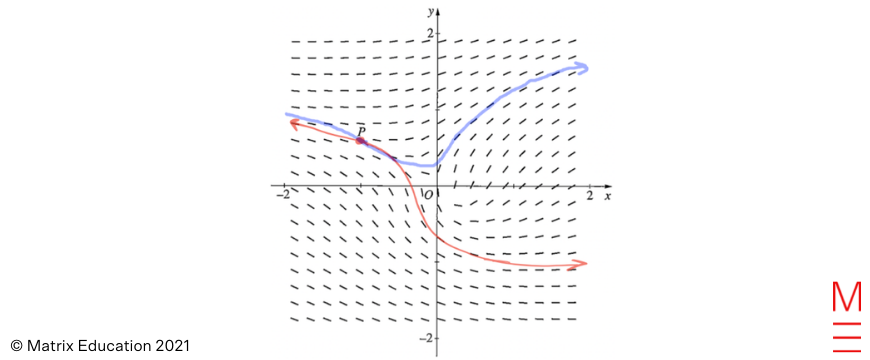### (i)

 We solve the differential equation by separating variables and integrating both sides. \begin{align*} \frac{dT}{T-25} &= k \,dt \\ \int \frac{1}{T-25} \,dT &= k \int \,dt \\ \ln|T-25| &= kt + C. \end{align*} Initially ($$t=0$$), the water is at $$5 ° \textrm{C}$$, so $$T-25 < 0$$ and we flip the terms in the absolute value. Substituting this point yields: \begin{align*} %\ln(25-T) = kt+C \overset{(t=0, T=5)}{\Longrightarrow} C = \ln 20. \ln(25-T) &= kt+C \\ \ln(25-5) &= k\times 0+C \\ C &= \ln 20. \end{align*} Now we can find $$k$$. \begin{align*} \ln(25-T) &= kt + \ln 20 \\ kt &= \ln(25-T) – \ln 20 = \ln\left(\frac{25-T}{20}\right). \qquad (*) \end{align*} At $$t=8$$, we are given that $$T=10$$. Hence \begin{equation*} 8k = \ln\left(\frac{15}{20}\right) = \ln\left(\frac{3}{4}\right) ⇒ k = \frac{1}{8}\ln\left(\frac{3}{4}\right). \end{equation*} We keep writing $$k$$ until the last step of the calculation in order to avoid propagating round-off errors. Now we determine the solution to the differential equation. \begin{align*} kt &= \ln\left(\frac{25-T}{20}\right) \\ e^{kt} &= \frac{25-T}{20} \\ 20e^{kt} &= 25-T \\ ⇒ T(t) &= 25 – 20e^{kt}. \end{align*} Substituting $$k$$ in and solving for $$T=20$$, we have: \begin{align*} 20 &= 25 – 20e^{\frac{1}{8}\ln(\frac{3}{4})t}\\ \frac{-5}{-20} &= e^{\frac{1}{8}\ln(\frac{3}{4})t}\\ \ln\left(\frac{1}{4}\right) &= \frac{1}{8}\ln\left(\frac{3}{4}\right)t\\ t &= \frac{\ln(\frac{1}{4})}{\frac{1}{8}\ln(\frac{3}{4})}\\ &= 38.55 \approx 39 \ \textrm{min.} \end{align*} A more efficient method: To find the time at which $$T=20$$, we may return to the earlier step $$(*)$$: \begin{equation*} kt = \ln\left(\frac{25-20}{20}\right) = \ln\left(\frac{5}{20}\right) = \ln\left(\frac{1}{4}\right). \end{equation*} Therefore \begin{equation*} t = \frac{1}{k}\ln\left(\frac{1}{4}\right) = 8 \frac{\ln(\tfrac{1}{4})}{\ln(\frac{3}{4})} =38.5507\ldots \approx 39 \ \text{min} \end{equation*} to the nearest minute.

### (ii)

We plot the function $$T(t)$$ below, showing the horizontal asymptote.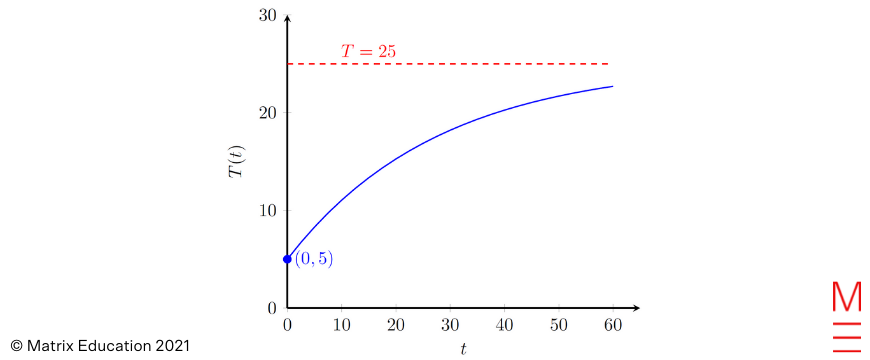### Question 12c

 If $$n=1$$, then the right hand side is\begin{equation*} \frac{1}{4} – \frac{1}{2\times 2\times 3} = \frac{2}{3\times 4} = \frac{1}{6} \end{equation*}while the left hand side is simply $$\frac{1}{1\times 2\times 3} = \frac{1}{6}$$. Hence the base case is true.Now assume the statement holds for some integer $$n\ge 1$$. We need to prove that\begin{equation*} \frac{1}{1\times 2\times 3} + \frac{1}{2\times 3\times 4} + \ldots + \frac{1}{n(n+1)(n+2)} + \frac{1}{(n+1)(n+2)(n+3)} = \frac{1}{4} – \frac{1}{2(n+2)(n+3)} \quad (\star) \end{equation*}Starting with the left hand side, we obtain\begin{multline*} \frac{1}{1\times 2\times 3} + \frac{1}{2\times 3\times 4} + \ldots + \frac{1}{n(n+1)(n+2)} + \frac{1}{(n+1)(n+2)(n+3)} \\ = \frac{1}{4} – \frac{1}{2(n+1)(n+2)} + \frac{1}{(n+1)(n+2)(n+3)} \end{multline*}by the induction assumption. Now we calculate \begin{align*} – \frac{1}{2(n+1)(n+2)} + \frac{1}{(n+1)(n+2)(n+3)} &= \frac{-(n+3) + 2}{2(n+1)(n+2)(n+3)} \\ &= \frac{-(n+1)}{2(n+1)(n+2)(n+3)} \\ &= \frac{-1}{2(n+2)(n+3)}. \end{align*} Therefore equation ($$\star$$) holds, which completes the induction step.

### (i)

We may first consider the basic parabola defined by $$y = (1-\frac{x}{2})^2$$ for $$x$$ in the domain $$(-\infty, 2]$$, which looks like this: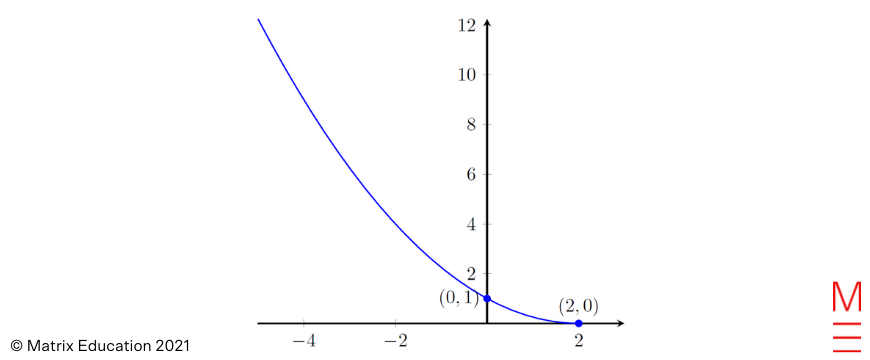Then $$y = 4-(1-\frac{x}{2})^2$$ is obtained by reflecting the above graph about the $$x$$-axis, and then shifting the vertex of the parabola up by 4 units: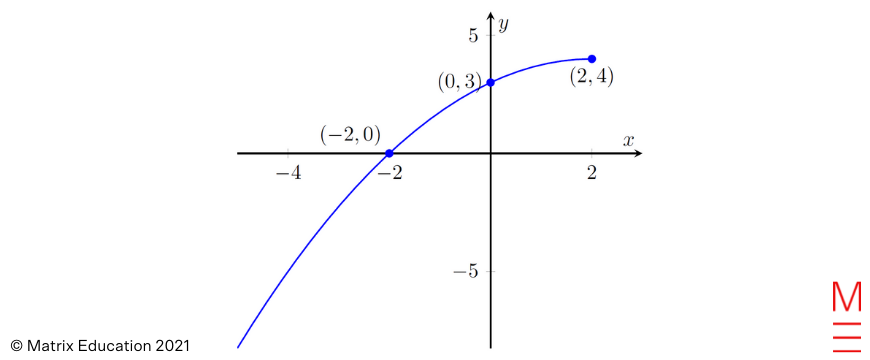### (ii)

 We consider the function $$f(x) = 4-(1-\frac{x}{2})^2$$ with domain $$(-\infty,2]$$ and range $$(-\infty, 4]$$. Thus the domain of the inverse function $$f^{-1}(x)$$ is $$(-\infty, 4]$$. Now we can determine $$f^{-1}$$ by first writing $$x = 4-(1-\frac{y}{2})^2$$, and then solve for $$y$$: \begin{align*} \left(1-\frac{y}{2}\right)^2 &= 4-x \\ 1-\frac{y}{2} &= \pm \sqrt{4-x} \\ \frac{y}{2} &= 1 \mp \sqrt{4-x} \\ ⇒ y &= 2 \mp 2\sqrt{4-x}. \end{align*} Finally we need to determine the correct choice of sign. Since $$f$$ passes through the point $$(x,y) = (-2,0)$$, it follows that $$f^{-1}$$ passes through $$(0,-2)$$. Plugging in $$x=0$$ into $$2 \mp 2\sqrt{4-x}$$, we find that $$–$$ is correct. Hence the inverse function is \begin{equation*} f^{-1}(x) = 2 – 2\sqrt{4-x} \end{equation*} with domain $$(-\infty, 4]$$.

### (iii)

We sketch $$f^{-1}$$ (red) along with $$f$$.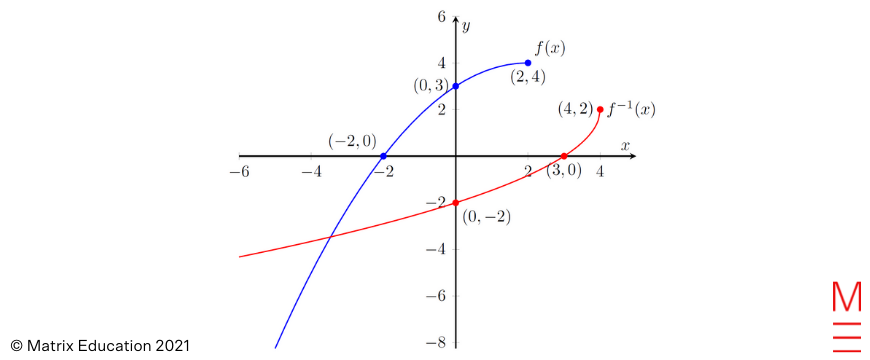### Question 13a

 The volume of the sculpture is calculated as the difference in volume between two paraboloids. The larger paraboloid, formed by the curve $$y=x^2$$ for $$0 \leq y \leq 2$$, has volume\begin{equation*} V_1 = \pi \int_0^2 x^2 \,dy = \pi \int_0^2 y \,dy = \pi \left[ \frac{y^2}{2} \right]^2_0 = 2\pi. \end{equation*}On the other hand, the smaller paraboloid is formed by the curve $$y=x^2+1$$ for $$1 \leq y \leq 2$$. We notice that $$x^2 = y-1$$ in this case, hence the volume is given by\begin{equation*} V_2 = \pi \int_1^2 x^2 \,dy = \pi \int_1^2 y-1 \,dy = \pi \left[ \frac{y^2}{2}-y \right]^2_1 = \frac{\pi}{2}. \end{equation*}Therefore \begin{equation*} V_{\text{sculpture}} = V_1 – V_2 = 2\pi – \frac{\pi}{2} = \frac{3\pi}{2} \text{ units}^3. \end{equation*}

### Question 13b

 We are given the following initial data: Initial velocity $$V=12 \text{ms}^{-1}$$; Launch angle $$\theta = 30 °$$; and Initial height $$h=1$$. Hence we find that $$Vt \cos\theta = 6\sqrt{3}\, t$$ and $$Vt\sin\theta = 6t$$. The trajectory of the ball is therefore parametrised by \begin{align*} x(t) &= 6\sqrt{3}\,t \\ y(t) &= -5t^2 + 6t + 1. \end{align*} We can find the maximum height already by differentiating $$y(t)$$. \begin{equation*} \dot{y}(t) = -10t + 6 = 0 ⇒ t = \frac{3}{5} \text{s}. \end{equation*} This is a maximum, since $$\ddot{y}(t) = -10 < 0$$. Hence \begin{equation*} y_{\max} = -5 \left( \frac{3}{5} \right)^2 + 6\left( \frac{3}{5} \right) + 1 = \frac{-9 + 18 + 5}{5} = \frac{14}{5}, \end{equation*} and we see that $$y_{\max} = 2.8 < 3$$. Hence the ball does not hit the ceiling. Now if we set $$x(t) = 10$$, then $$6\sqrt{3} t = 10$$, which gives \begin{equation*} t = \frac{5}{3\sqrt{3}} \text{s}. \end{equation*} This is the time at which the ball hits the far wall. However, we check on our calculator that \begin{equation*} y\left( \frac{5}{3\sqrt{3}} \right) = -5\left( \frac{5}{3\sqrt{3}} \right)^2 + 6\left( \frac{5}{3\sqrt{3}} \right) + 1 \approx 2.144 > 0 \end{equation*} (where we have given the result correct to 3 decimal places). This shows the vertical displacement is positive, so we can deduce that the ball has not yet hit the ground.

### Question 13c

 We apply the area bounded between two curves formula, noting that we have an even function and so we can simplify things by only finding the area from $$x=0$$ to $$x=2$$ and doubling our answer. \begin{align*} A &= \int_{-2}^{2} 2 – |x| – \left(1-\frac{8}{4+x^2}\right) \ \mathrm{d} x\\ &= 2 \int_0^2 1-x+\frac{8}{4+x^2} \; \mathrm{d}x\\ &= 2 \left[x-\frac{1}{2} x^2 + 4\tan^{-1} \frac{x}{2}\right]_0^2 \\ &= 2\pi \ \mathrm{units}^2. \end{align*}

### (i)

 If $$A=B-d$$ and $$C=B+d$$ then \begin{align*} \frac{\sin A+\sin C}{\cos A + \cos C} &= \frac{\sin(B-d)+\sin(B+d)}{\cos(B-d)+\cos(B+d)} \\ &= \frac{2\sin B \cos d}{2\cos B \cos d} \\ &= \tan B \end{align*} where we make the use of the sum to product formulae in the first step.

### (ii)

 If we let $$B=\frac{11\theta}{14}$$ and $$d=\frac{\theta}{14}$$ in (i) then we get:\begin{equation*} \frac{\sin \frac{5\theta}{7} + \sin \frac{6\theta}{7}}{\cos \frac{5\theta}{7} + \cos \frac{6\theta}{7}} = \tan \frac{11\theta}{14} \end{equation*}and so we simplify our problem to solving the equation\begin{equation*} \tan \frac{11\theta}{14} = \sqrt{3} \qquad \mathrm{where} \qquad 0\leq \theta \leq 2\pi ⇒0\leq \frac{11\theta}{14}\leq \frac{11\pi}{7}. \end{equation*}Looking at first and third quadrant solutions, we obtain\begin{align*} \frac{11\theta}{14} &= \frac{\pi}{3}, \frac{4\pi}{3} \\ ∴\theta &= \frac{14\pi}{33}, \frac{56\pi}{33} \end{align*}

### Question 14a

 Consider the following diagram, where the 175km/h vector is placed tip-to-tail with the 42 km/h wind vector. By alternate angles, the angle between the wind vector and the destination vector is $$63^\circ$$.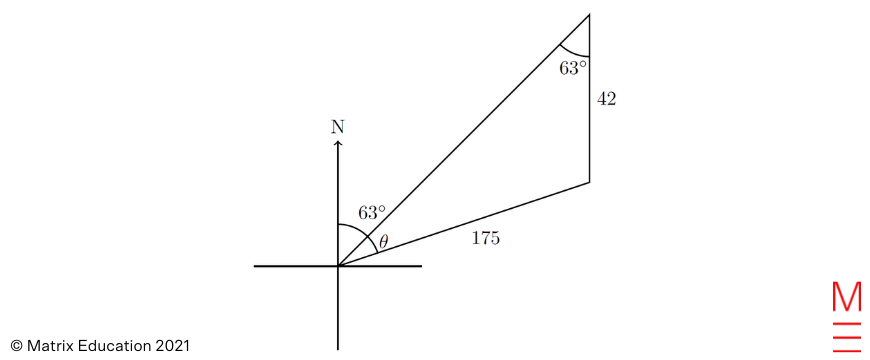Using the sine law, \begin{align*} \frac{\sin \theta}{42} &= \frac{\sin 63^\circ}{175} \\ \theta &= \sin^{-1}\left(\frac{42 \sin 63^\circ}{175}\right) \\ &\approx 12^\circ \; \text{(nearest degree)} \end{align*} Hence, the bearing is $$063^\circ T + \theta = 075^\circ T$$ to the nearest degree.

### Question 14b

 This is a classic example of a logistic equation. We first separate variables\begin{equation*} \frac{C}{P(C-P)} \,dP = 0.1\,dt \end{equation*}and then integrate both sides, using the given fact. Note that we use $$K$$ for our integration constant, since $$C$$ is already taken!\begin{align*} \int \frac{C}{P(C-P)} \,dP &= \int 0.1 \,dt = 0.1t + K \\ ⇒ 0.1t + K &= \int \frac{1}{P} + \frac{1}{C-P} \,dP \\ &= \ln P – \ln(C-P) = \ln\left(\frac{P}{C-P}\right). \end{align*}(We do not need absolute values here: we must have $$C \ge P(t)$$ for all $$t\ge 0$$, since $$C$$ is the carrying capacity, i.e. the largest population the habitat can sustain).At $$t=0$$ (the year 1980), we are given that the deer population was $$150\, 000$$. Setting $$t=0$$ and $$P=150\, 000$$ in the above result, we find\begin{equation*} K = \ln\left( \frac{150\, 000}{C – 150\, 000} \right). \end{equation*}In the year 2000, which corresponds to $$t=20$$, we are given that $$P = 600\, 000$$. Therefore \begin{equation*} 2 + K = \ln\left( \frac{600\, 000}{C-600\, 000} \right). \end{equation*} We can now eliminate $$K$$ and start solving for $$C$$: \begin{align*} K = \ln\left( \frac{150\, 000}{C – 150\, 000} \right) &= \ln\left( \frac{600\, 000}{C-600\, 000} \right) – 2 \\ \ln\left( \frac{600\, 000}{C-600\, 000} \right) – \ln\left( \frac{150\, 000}{C – 150\, 000} \right) &= 2 \\ \ln\left(\frac{600\,000}{C – 600\, 000} \cdot \frac{C – 150\, 000}{150\, 000} \right) &= 2 \\ \ln\left( 4\frac{C-150\,000}{C-600\,000}\right) = 2. \end{align*} Taking the exponential of both sides, we continue to solve: \begin{align*} 4\frac{C-150\,000}{C-600\,000} &= e^2 \\ \frac{C-150\,000}{C-600\,000} &=\frac{1}{4}e^2 \\ C – 150\,000 &= \frac{1}{4} e^2(C – 600\,000) \\ \left(\frac{1}{4}e^2 – 1\right)C &= 150\, 000(e^2 – 1) \\ ⇒ C &= 150\,000 \frac{e^2 -1}{\frac{1}{4}e^2 – 1} \approx 1\, 131\, 121. \end{align*} The carrying capacity is therefore $$C \approx 1\, 130\, 000$$, to 3 significant figures.

### (i)

 The easiest way to do this is algebraically. Let $$v= x \underset{\text{~}}{i} + y\underset{\text{~}}{j}$$ then $$\underset{\text{~}}{v}\cdot\underset{\text{~}}{v} = x^2+y^2.$$ We now check the right hand side, $$|\underset{\text{~}}{v}|^2 = \left(\sqrt{x^2+y^2}\right)^2=x^2+y^2$$ and so we conclude that $$\underset{\text{~}}{v}\cdot\underset{~}{v} = |\underset{\text{~}}{v}|^2$$.

### (ii)

 Note that\begin{equation*} \overrightarrow{AC} = \overrightarrow{AB}+\overrightarrow{BC} = \underset{\text{~}}{a}+\underset{\text{~}}{b} \qquad \mathrm{and} \qquad \overrightarrow{BD} = \overrightarrow{AD}-\overrightarrow{AB} = k \underset{\text{~}}{b}-\underset{\text{~}}{a} \end{equation*}Using the fact that $$|\overrightarrow{AC}| = |\overrightarrow{BD}|$$, or rather (in order to use (i)), $$|\overrightarrow{AC}|^2 = |\overrightarrow{BD}|^2$$ to obtain\begin{equation*} \overrightarrow{AC}\cdot\overrightarrow{AC} = \overrightarrow{BD}\cdot\overrightarrow{BD} \end{equation*}Substituting for our expressions for $$\overrightarrow{AC}$$ and $$\overrightarrow{BD}$$ and rearranging, we get\begin{equation*} (\underset{\text{~}}{a}+\underset{\text{~}}{b})\cdot(\underset{\text{~}}{a}+\underset{\text{~}}{b}) = (k\underset{\text{~}}{b}-\underset{\text{~}}{a})\cdot(k\underset{\text{~}}{b}-\underset{\text{~}}{a}) \end{equation*}Now we expand both sides and make use of (i) to obtain the required result:\begin{align*} \underset{\text{~}}{a}\cdot \underset{\text{~}}{a}+2\underset{\text{~}}{a}\cdot \underset{\text{~}}{b}+\underset{\text{~}}{b}\cdot\underset{\text{~}}{b}&=k^2\underset{\text{~}}{b}\cdot\underset{\text{~}}{b}-2k\underset{\text{~}}{a}\cdot\underset{\text{~}}{b}+\underset{\text{~}}{a}\cdot\underset{\text{~}}{a} \\ 2\underset{\text{~}}{a}\cdot\underset{\text{~}}{b}+|\underset{\text{~}}{b}|^2&=k^2|\underset{\text{~}}{b}|^2-2k\underset{\text{~}}{a}\cdot \underset{\text{~}}{b}\\ 2(1+k)\underset{\text{~}}{a}\cdot\underset{\text{~}}{b} &= (k^2-1)|\underset{\text{~}}{b}|^2 \\ 2(1+k)\underset{\text{~}}{a}\cdot\underset{\text{~}}{b} &= -(1+k)(1-k)|\underset{\text{~}}{b}|^2 \\ 2\underset{\text{~}}{a}\cdot\underset{\text{~}}{b} &= -(1-k)|\underset{\text{~}}{b}|^2 \quad \text{since } 1+k \neq 0\\ 2\underset{\text{~}}{a}\cdot\underset{\text{~}}{b}+(1-k)|\underset{\text{~}}{b}|^2 &= 0. \end{align*}

### Question 14d

 With $$p = \frac{3}{500}$$, we can calculate the following quantities for $$\hat p$$,\begin{align*} \mu &= p = \frac{3}{500} \\ \sigma^2 &= \frac{p(1-p)}{n} = \frac{1491}{500^2 n} \end{align*}The aim is to find the probability $$P(\hat p \geq \frac{4}{500})$$, which we will change into z-scores,\begin{align*} P(\hat p \geq \frac{4}{500}) &= P \left(Z \geq \frac{\frac{4}{500}-\mu}{\sigma}\right)\\ &= P \left(Z \geq \frac{\frac{4}{500} – \frac{3}{500}}{\frac{1}{500} \sqrt{\frac{1491}{n}}} \right) \\ &= P \left(Z \geq \frac{\sqrt{n}}{\sqrt{1491}} \right) \end{align*}From the empirical rule, 95% of the data is between z-scores -2 and 2.So, 2.5% is to the right of 2.Hence, we need\begin{align*} \frac{\sqrt{n}}{\sqrt{1491}} &\geq 2 \\ n &\geq 4 \times 1491 = 5964. \end{align*} Hence, the sample size required is 6000 to the nearest thousand.

### Question 14e

 We have that $$g(x)=x^3+4x-2$$ and $$g'(x)=3x^2+4$$. In order to find the gradient of the tangent to $$f(x)=x g^{-1}(x)$$ at $$x=3$$ we need to find $$f'(3)$$. Differentiating $$f(x)$$ using the product rule, we get\begin{align*} f'(x) = g^{-1}(x) + x \frac{d}{dx}\left(g^{-1}(x)\right) \end{align*}It becomes necessary to differentiate $$g^{-1}(x)$$. To find what this is, consider the function $$y=g^{-1}(x)$$: we have $$x=g(y)$$, and we want to find $$\frac{dy}{dx}$$.Then\begin{align*} \frac{dx}{dy} &= g'(y) \\ \frac{dy}{dx} &= \frac{1}{g'(y)} \end{align*}However, note here that $$y$$ in this context is not the same as $$g(x)$$, because in this context, $$y = g^{-1}(x)$$. We should instead try to express $$\frac{dy}{dx} = \frac{d}{dx}(g^{-1}(x))$$ in terms of $$x$$ only, by substituting $$y = g^{-1}(x)$$:\begin{align*} \frac{dy}{dx} = \frac{d}{dx} (g^{-1}(x))&= \frac{1}{g'(y)} \\ &= \frac{1}{g’\left(g^{-1}(x)\right)} \end{align*}So the derivative of $$f(x)$$ is given by \begin{equation*} f'(x) = g^{-1}(x) + \frac{x}{g’\left(g^{-1}(x)\right)} \end{equation*} Since $$g(x)$$ passes through the point $$(1,3)$$, we have $$g(1)=3$$ so $$g^{-1}(3)=1$$. Now substituting $$x=3$$ we have \begin{align*} f'(3) &= g^{-1}(3) + \frac{3}{g’\left(g^{-1}(3)\right)} \\ &= 1 + \frac{3}{g'(1)} \\ &= 1 + \frac{3}{3\times1^2+4} \\ &= \frac{10}{7} \end{align*} We conclude that the gradient of the tangent to $$f(x)$$ at $$x=3$$ is $$\frac{10}{7}$$.